nextnano.com  GUI: nextnanomat  Tool: nextnano++  Tool: nextnano³  Tool: nextnano.NEGF  Download | Search | Copyright | News | Publications  * password protected nextnano³ software1D SL minibands

# nextnano3 - Tutorial

## Dispersion in infinite superlattices: Minibands (Kronig-Penney model)

Author: Stefan Birner

```-> 1Dsuperlattice_dispersion_4nm_nn3.in       / *_nnp.in - ```input file for the nextnano3 and nextnano++ software``` -> 1Dsuperlattice_dispersion_6nm_nn3.in       / *_nnp.in - ```input file for the nextnano3 and nextnano++ software``` -> 1Dsuperlattice_dispersion_bulk_GaAs_nn3.in / *_nnp.in - ```input file for the nextnano3 and nextnano++ software``` -> Superlattice_1D_nn3.in                     / *_nnp.in - ```input file for the nextnano3 software

`==> ` Download these input files
`    `If you don't have a password yet, you have to first sign up for a free evaluation license in order to download these input files.

## Dispersion in infinite superlattices: Minibands (Kronig-Penney model)

This tutorial aims to reproduce two figures (Figs. 2.27, 2.28, p. 56f) of Paul Harrison's excellent book "Quantum Wells, Wires and Dots", thus the following description is based on the explanations made therein.
We are grateful that the book comes along with a CD so that we were able to look up the relevant material parameters and to check the results for consistency.

### Superlattice 1:  4 nm AlGaAs / 4 nm GaAs

• Our infinite superlattice consists of a 4 nm GaAs quantum well surrounded by 2 nm Al0.4Ga0.6As barriers on each side. The choice of periodic boundary conditions leads to the following sequence of identical quantum wells: 4 nm AlGaAs / 4 nm GaAs / 4 nm AlGaAs / 4 nm GaAs / ... . So our superlattice period has the length L=8 nm.
(Actually it has the length L = 8.25 due to the grid point resolution of 0.25 nm.)

This figure shows the conduction band edge and the first eigenstate that is confined inside the well and its corresponding charge density (psi²)  for the superlattice vector kz = 0. Note that periodic boundary conditions are employed for solving the Schrödinger equation. The second eigenstate is not confined inside the well and is therefore not shown here.
(Note that the energies were shifted so that the conduction band edge of GaAs equals 0 eV.)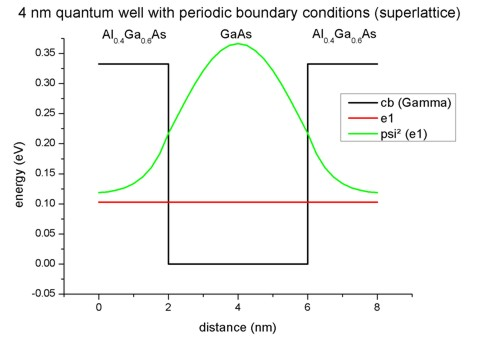• In a superlattice the electrons (and holes) see a periodic potential which is similar to the periodic potential in bulk crystals. This means that the particle wave functions are no longer localized in one quantum well. They extend to infinity and they are equally likely to be found in any of the quantum wells. The eigenstates are called Bloch states (as in bulk) and the wave functions are periodic:
Psi (z) = Psi (z + L)
For a travelling wave of the form exp(ikzz) it holds:
Psi (z + L) = exp(ikz(z + L)) = exp(ikzz) exp(ikzL)
i.e. Psi (z + L) = Psi (z) exp(ikzL)
kz is the momentum of the electron (or hole) along the growth direction of the infinite superlattice.
• Here we plot the superlattice dispersion curve, i.e. the energy of the electron as a function of its superlattice wave vector kz for the lowest eigenstate. As the energy is a periodic function of kz with period 2pi/L, we plot only the interval [ - pi / L , + pi / L].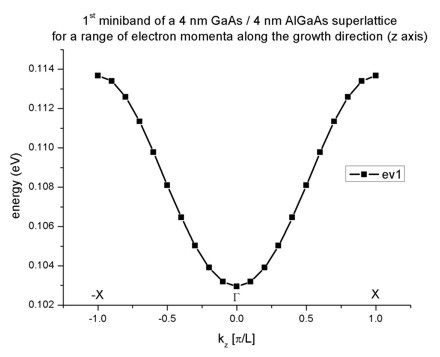The plot is in excellent agreement with Fig. 2.27 (page 56) of Paul Harrison's book "Quantum Wells, Wires and Dots".
When the electron is at rest (kz=0), the dispersion curve shows a minimum. As the electron momentum kz increases, its energy also increases and reaches a maximum at k = - pi/L and k = + pi/L. Thus the electron within the superlattice occupies a continuum of energies. This continuum that is bound by a maximum and a minimum of energy is called miniband. Due to the similarity with the energy bands of a bulk crystal, the point in the superlattice Brillouin zone for kz=0 is called Gamma and for kz=pi/L it is called X.

### Superlattice 2:  6 nm AlGaAs / 6 nm GaAs

• Our second infinite superlattice consists of a 6 nm GaAs quantum well surrounded by 3 nm Al0.4Ga0.6As barriers on each side. The choice of periodic boundary conditions leads to the following sequence of identical quantum wells: 6 nm AlGaAs / 6 nm GaAs / 6 nm AlGaAs / 6 nm GaAs / ... . So our superlattice period has the length L=12 nm.
(Actually it has the length L = 12.25 due to the grid point resolution of 0.25 nm.)

This figure shows the conduction band edge and the two lowest eigenstates that are confined inside the well and their corresponding charge density (psi²)  for the superlattice vector kz = 0. Note that periodic boundary conditions are employed for solving the Schrödinger equation. The third eigenstate is not confined inside the well and is therefore not shown here.
In contrast to the 4 nm quantum well superlattice described above, two confined electron states exist.
(Note that the energies were shifted so that the conduction band edge of GaAs equals 0 eV.)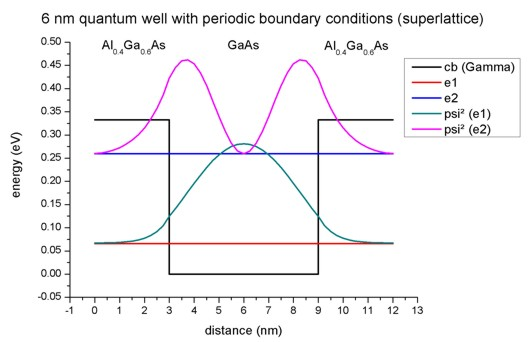• The following figure shows the first two minibands for this superlattice. They arise from the first and the second eigenstate. Note that due to the scale of this figure the first miniband looks almost flat. It is also interesting that for the second miniband the minimum is not at the center (i.e. at Gamma) but at the edges of the superlattice Brillouin zone at X (and -X).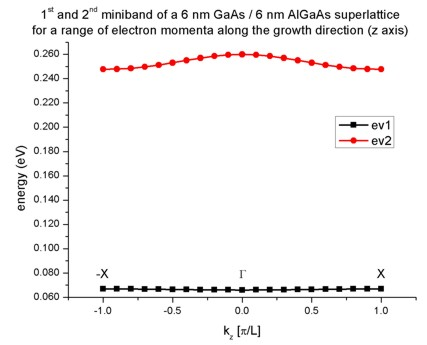Again, the plot is in excellent agreement with Fig. 2.28 (page 57) of Paul Harrison's book "Quantum Wells, Wires and Dots". However, the caption of Fig. 2.28 incorrectly states that this should be a 8 nm GaAs / 8 nm Al0.4Ga0.6As superlattice. In fact, it must be a 6 nm GaAs / 6 nm Al0.4Ga0.6As superlattice.

## Technical details

• The resolution of the miniband plot has to be specified within the keyword``` \$quantum-model-electrons```:
` \$quantum-model-electrons`
```  ...   boundary-condition-001 = periodic ! ```periodic boundary conditions are necessary for superlattices```   num-ks-001             = 21       ! ``` number of superlattice vectors along z direction``` ```For each superlattice vector kz, the Schrödinger equation has to be solved. The 11th superlattice vector corresponds to kz=0 which is obviously identical to the case when no superlattice is specified at all.

The miniband dispersion is written to this file:
```  Schroedinger_1band/sg_dispSL1D_el_qc001_sg001_deg001_evmin001_evmax002.dat ```It contains the following data:
```  k_z [pi/L]        k_z [1/AA]      1st eigenvalue      2nd eigenvalue   -1.0              ...             ...                ...```

## Dispersion in bulk GaAs with periodic boundary conditions

We take the same input file as``` 1Dsuperlattice_dispersion_6nm_nn3.in ```but this time we replace the AlGaAs barrier with GaAs so that we have only pure bulk GaAs with a length of 12 nm. So our superlattice period has the length L=12 nm.
(Actually it has the length L = 12.25 due to the grid point resolution of 0.25 nm.)
At the boundaries we apply periodic boundary conditions and the same superlattice options as above:

```1Dsuperlattice_dispersion_bulk_GaAs_nn3.in: ```
` \$quantum-model-electrons`
```  ...   boundary-condition-001 = periodic ! ```periodic boundary conditions are necessary for superlattices```   num-ks-001             = 21       ! ``` number of superlattice vectors along z direction

This figure shows the conduction band edge and the three lowest eigenstates and their corresponding charge density (psi²) for the superlattice vector kz = 0. Note that periodic boundary conditions are employed for solving the Schrödinger equation.
- The ground state wave function is constant with its energy equal to the conduction band edge energy.
- The energies of the second and third eigenstate are degenerate.
(Note that the energies were shifted so that the conduction band edge of GaAs equals 0 eV.)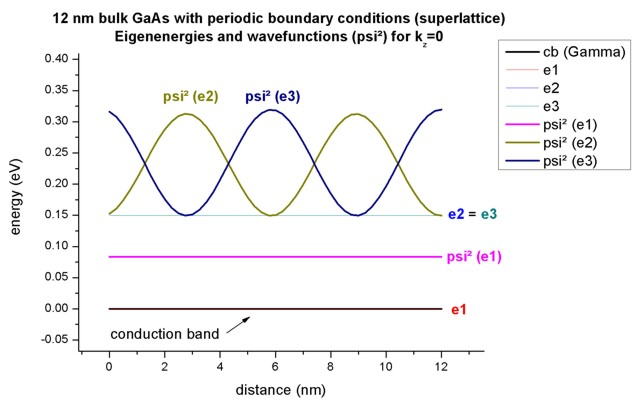The following figure shows the first three minibands for this superlattice. They arise from the first, second and third eigenstate.
The second and third eigenstate are degenerate at kz = 0 as can be seen also in the figure above. Also at kz = -1 and kz = 1, the first and second eigenstate are degenerate. This is as expected because the dispersion should look like the parabolic dispersion E(k) of bulk GaAs.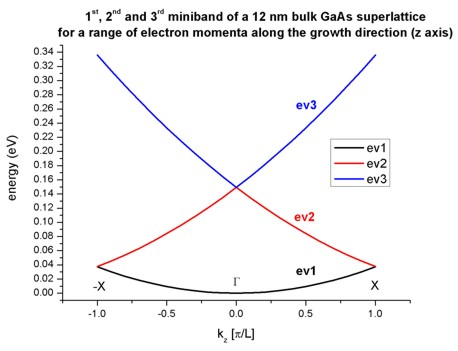• Please help us to improve our tutorial Send comments to``` support [at] nextnano.com```.

## Template

```-> Superlattice_1D_nn3.in                     / *_nnp.in - ```input file for the nextnano3 software

We want to study the energy levels of a superlattice in order to understand how they form bands in a periodic structure.
One can easily see this by calculating the energy levels for various barrier heights, i.e. we automatically generate input files for the variable "Barrier_Height".
Once done, we visualize the "band structure file" called``` sg_dispSL_el_sg1_deg1_piL_evmin001_evmax004.dat```.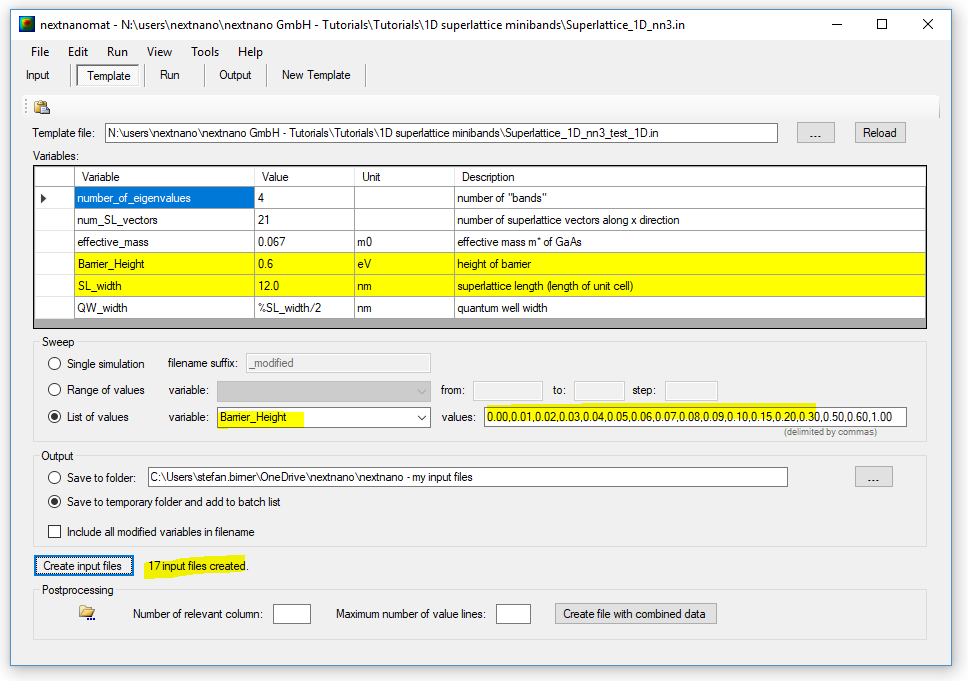The left figure contains a quantum well superlattice with a barrier height of 0 eV, i.e. a bulk semiconductor while the figure on the right shows the dispersion for a barrier height of 0.06 eV.
One can clearly see that three energy band gaps open.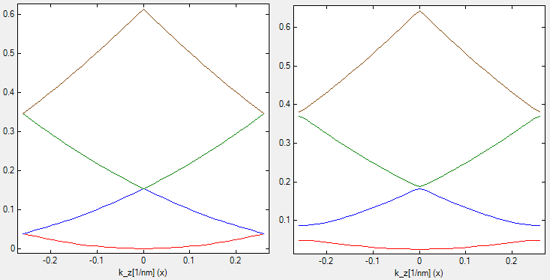• Please help us to improve our tutorial! Send comments to``` support [at] nextnano.com```.